# R syntactic sugar¶

SimpleITK in R attempts to make all the common R array operators and arithmetic work on images.

First we load SimpleITK and set up a viewer for notebooks, using the jet colourmap.

In :
library(SimpleITK)

Dwidth <- grid::unit(10, "cm")
## version of show using a jet colourmap.
setMethod('show', '_p_itk__simple__Image',
function(object)
{
jet.colors <- colorRampPalette(c("#00007F", "blue", "#007FFF", "cyan", "#7FFF7F", "yellow", "#FF7F00", "red", "#7F0000"))
a <- t(as.array(object))
rg <- range(a)
A <- (a-rg)/(rg-rg)
dd <- dim(a)
sp <- object$GetSpacing() sz <- object$GetSize()
worlddim <- sp*sz
worlddim <- worlddim/worlddim
W <- Dwidth
H <- Dwidth * worlddim
jc <- jet.colors(101)
A <- jc[A*100 + 1]
dim(A) <- dim(a)
grid::grid.raster(A,default.units="mm", width=W, height=H)

}

)
## Make a smaller plot size
options(repr.plot.height = 3, repr.plot.width=3)

In :
img = GaussianSource("sitkUInt8", size=c(64,64))
img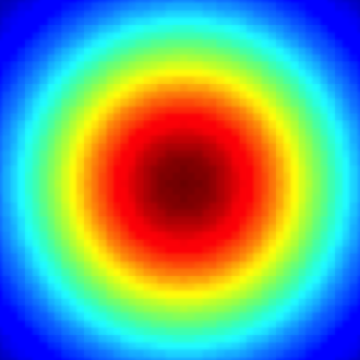Here we note a problem with the swig bindings to the procedural interface - dispatching and defaults are not as nice as the python version. This means that we can't say

GaborSource("sitkFloat32", size=c(64,64), frequency=.03)


because there are arguments missing before frequency and swig can't generate default arguments from c++ code.

## Image slicing and indexing¶

Lets start by accessing a single pixel - note that indexing starts from 1:

In :
img[25,25]

198

### Cropping¶

In :
img[17:49,]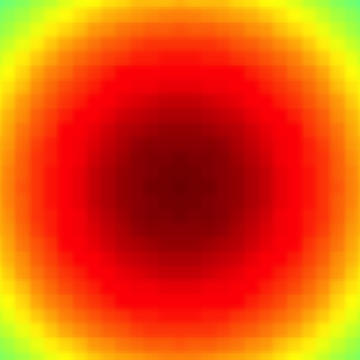In :
## Don't have the "from the end" slicing - negatives mean remove

img[,16:(img$GetHeight() - 16)]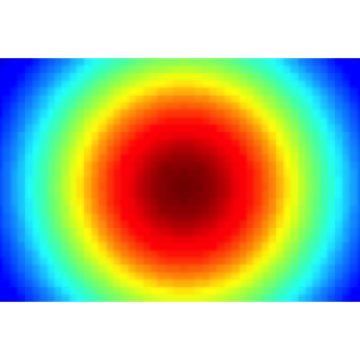In : img = GaborSource("sitkFloat32", size=c(64,64), sigma=c(16, 16), mean=c(32,32), frequency=.03) img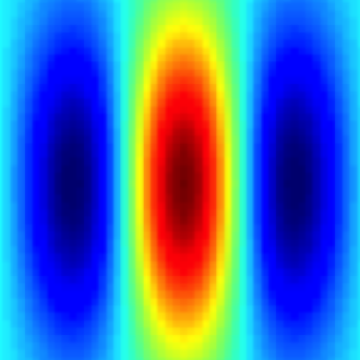In : img[, -(1:16)]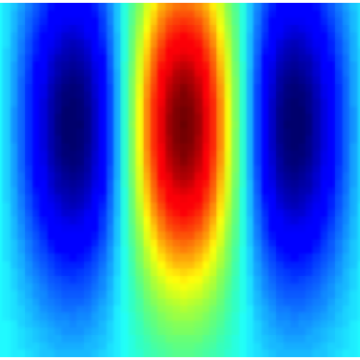In : img[1:32, 1:32]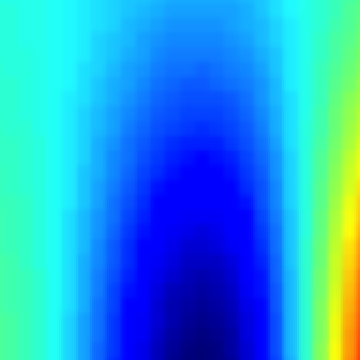### Flipping¶ In : corner=img[1:32, 1:32] corner[corner$GetWidth():1,]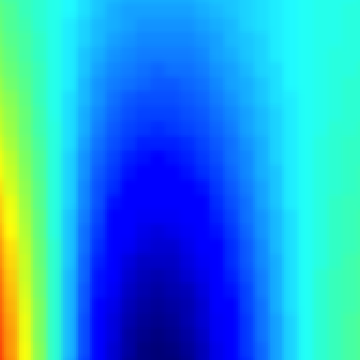### Tiling (using slicing)¶

We cannot perform tiling via slicing directly on an image. The reason for this restriction is that an image is not equivalent to an array. Unlike an array, an image enforces the concept of physical spacing between pixels/voxels, this spacing has to be uniform for each of the axes. Thus a non-uniform slicing, e.g. (1,2,3,3,2,1), will generate an error.

In :
corner[c(1:corner$GetWidth(), corner$GetWidth():1), c(1:corner$GetHeight(), corner$GetHeight():1)]

Error in corner[c(1:corner$GetWidth(), corner$GetWidth():1), c(1:corner$GetHeight(), : X spacing is not uniform Traceback: 1. corner[c(1:corner$GetWidth(), corner$GetWidth():1), c(1:corner$GetHeight(),
.     corner$GetHeight():1)] 2. corner[c(1:corner$GetWidth(), corner$GetWidth():1), c(1:corner$GetHeight(),